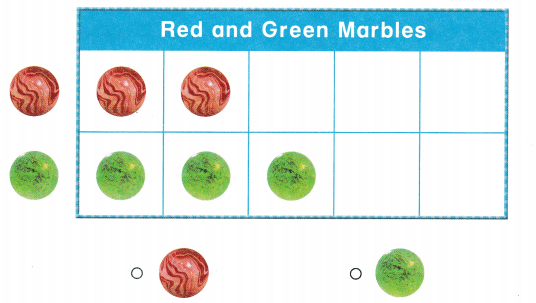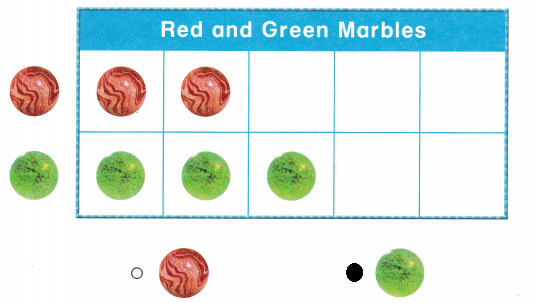# Texas Go Math Kindergarten Lesson 20.3 Answer Key Make and Read a Real-Object Graph

Refer to our Texas Go Math Kindergarten Answer Key Pdf to score good marks in the exams. Test yourself by practicing the problems from Texas Go Math Kindergarten Lesson 20.3 Answer Key Make and Read a Real-Object Graph.

## Texas Go Math Kindergarten Lesson 20.3 Answer Key Make and Read a Real-Object Graph

Essential Question
How can you make a real-object graph to count objects that have been sorted into categories?

Explore.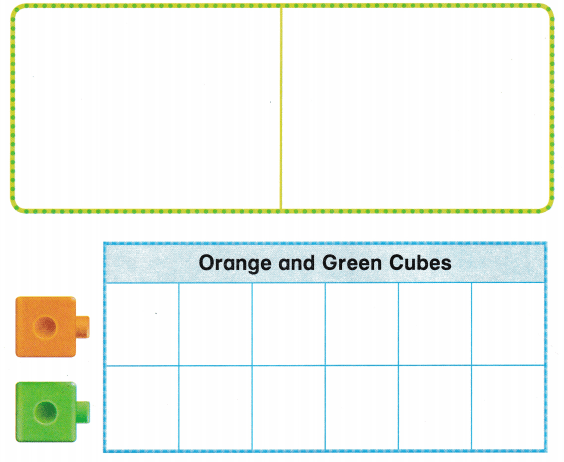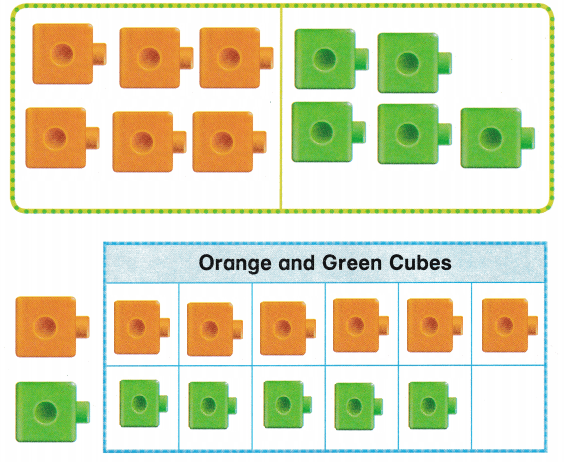Explanation:
There are 6 orange cubes
and 5 green cubes.
so, sorted according to the colors

Directions
Place a handful of orange and green cubes on the workspace, Sort and classify the cubes by color. Move the cubes to the graph. Draw and color the cubes, Tell a friend how many cubes are in each category.

Share and Show

Question 1.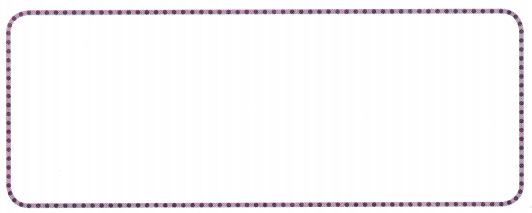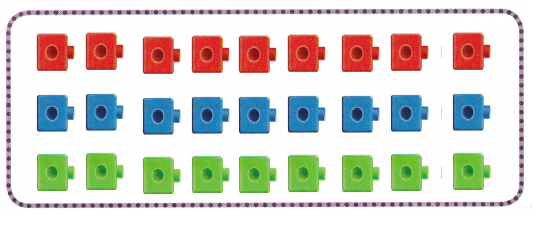Explanation:
On a work sheet
we have placed
9 red colored cubes,9 blue colored cubes and 9 green colored cubes.

Question 2.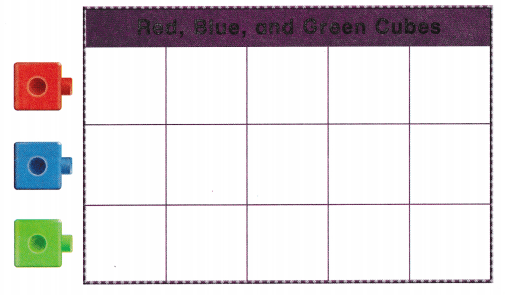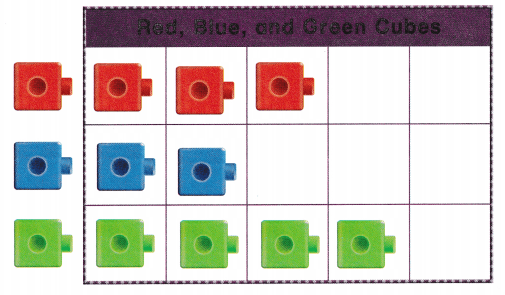Explanation:
There are 3 red cubes and 2 blue cubes
and 4 green cubes.

Question 3.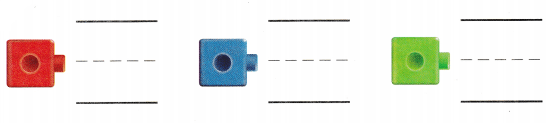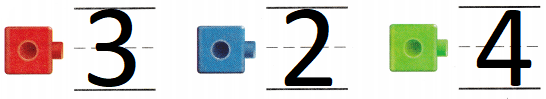Explanation:
There are 3 red cubes and 2 blue cubes
and 4 green cubes.

Directions
1. Place a handful of red, blue, and green cubes on the workspace. Sort the cubes by color. 2. Move the cubes to the graph. Draw and color the cubes. 3. Read the graph. Write how many of each cube.

Question 4.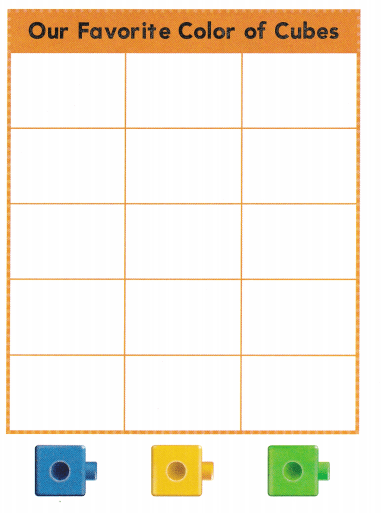Explanation:
There are 2 blue cubes
2 yellow cubes
and 1 green cube with the classmates.

Question 5.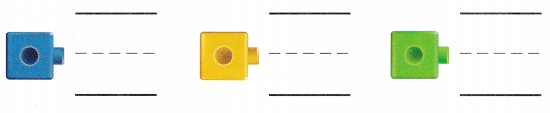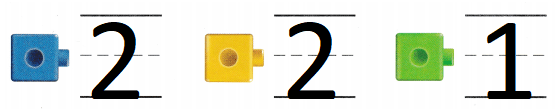Explanation:
There are 2 blue cubes
2 yellow cubes
and 1 green cube with the classmates.

Directions
4. Use blue, yellow, and green cubes. Ask 5 classmates to choose their favorite color cube. Place the cubes in the graph. Draw and color the cubes. 5. Read the graph. Write how many of each color.

Problem Solving

Question 6.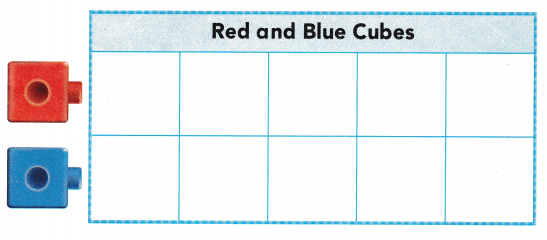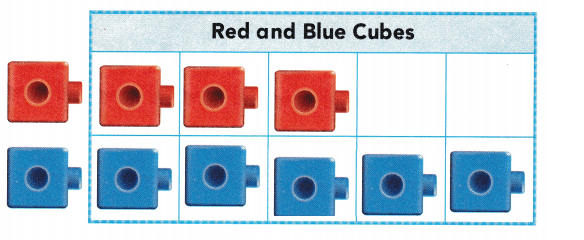Explanation:
Eli has 3 red cubes.
he has 2 more than red cubes
3 + 2 = 5
so, Eli has 5 blue cubes.

Question 7.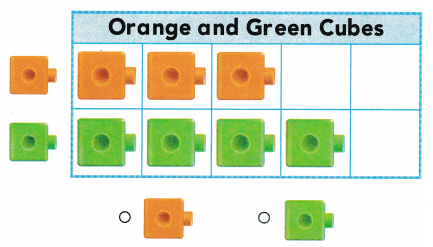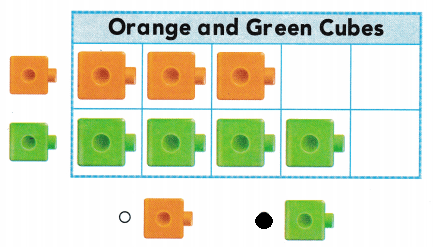Explanation:
There are 3 orange cubes and
4 green cubes
so, circled the number 4.

Directions
6. Eli has 3 red cubes. He has two more blue cubes than red cubes. Draw to show the cubes on the graph. 7. Choose the correct answer. Look at the graph. Are there more orange cubes or more green cubes?

### Texas Go Math Kindergarten Lesson 20.3 Homework and Practice

Question 1.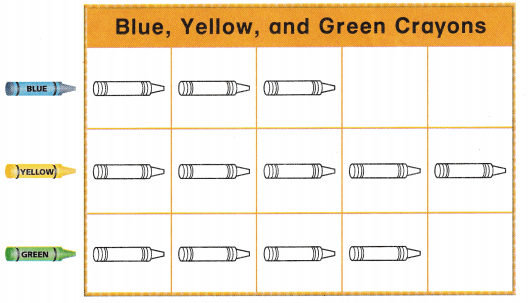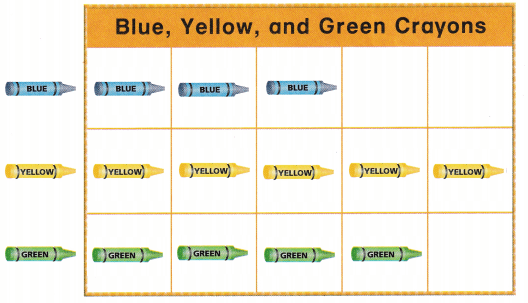Explanation:
In a crayon box there are 3 blue crayons
5 yellow crayons and 4 green crayons.

Question 2.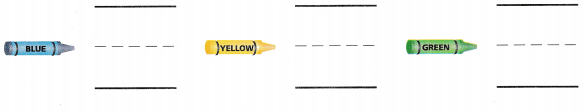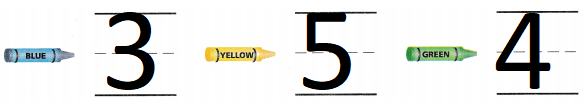Explanation:
In a crayon box there are 3 blue crayons
5 yellow crayons and 4 green crayons.

Directions Look at the graph. 1. In the top row, color the crayons blue. In the middle row, color the crayons yellow. In the bottom row, color the crayons green. 2. Read the graph. Write how many of each color.

Texas Test Prep

Lesson Check

Question 3.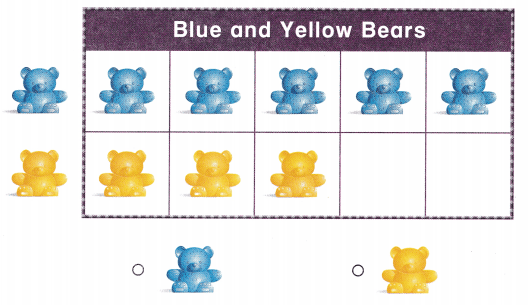Explanation:
Children has 5 blue bears and
3 yellow bears
as blue bears are more
so, marked the blue bears.

Question 4.